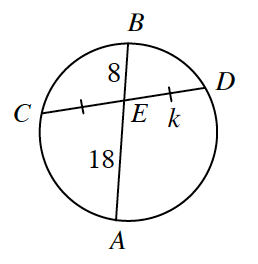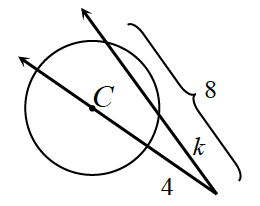### Home > CCG > Chapter 12 > Lesson 12.1.2 > Problem12-33

12-33.

Use all your circle relationships to solve for the variables in each of the diagrams below.

1. $\overline{AB}$ and $\overline{CD}$ intersect at $E$.$(CE) (ED) = (AE) (EB)$

$(k) (k) = (18) (8)$

1. The area of $\odot C$ is $25\pi$ sq. unitsBecause the area is $25\pi$, that means the radius is $5$, and the diameter is $10$.

$\begin{array}{l} \frac{10+4}{k}=\frac{8}{4} \\ 56=8k \\ k=7 \end{array}$